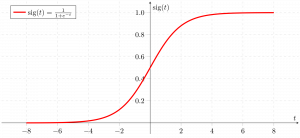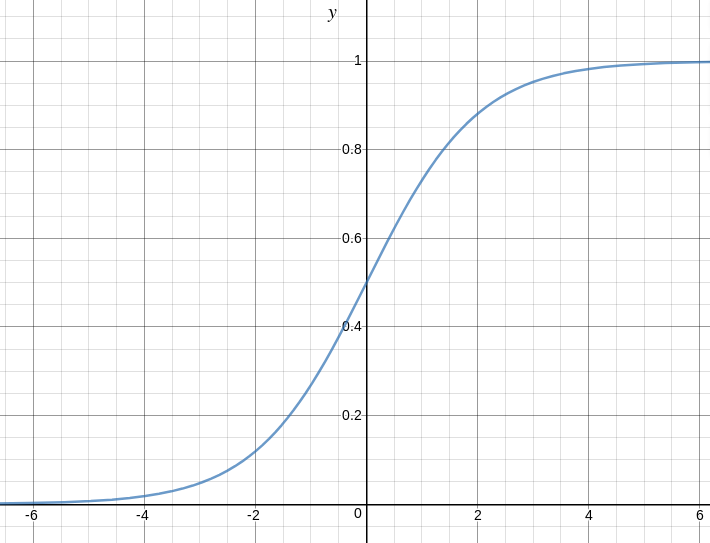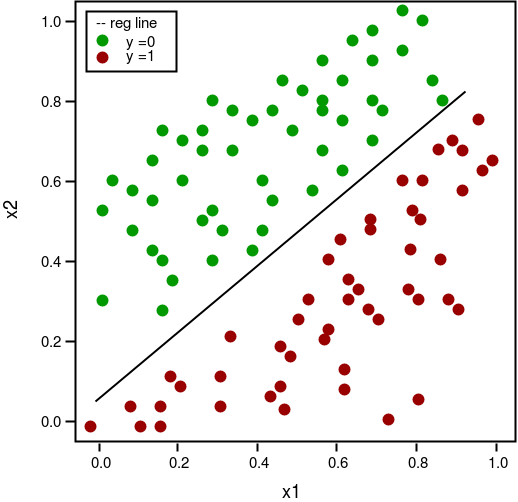# Understanding Logistic Regression

Pre-requisite: Linear Regression
This article discusses the basics of Logistic Regression and its implementation in Python. Logistic regression is basically a supervised classification algorithm. In a classification problem, the target variable(or output), y, can take only discrete values for given set of features(or inputs), X.

Contrary to popular belief, logistic regression IS a regression model. The model builds a regression model to predict the probability that a given data entry belongs to the category numbered as “1”. Just like Linear regression assumes that the data follows a linear function, Logistic regression models the data using the sigmoid function.Logistic regression becomes a classification technique only when a decision threshold is brought into the picture. The setting of the threshold value is a very important aspect of Logistic regression and is dependent on the classification problem itself.

The decision for the value of the threshold value is majorly affected by the values of precision and recall. Ideally, we want both precision and recall to be 1, but this seldom is the case. In case of a Precision-Recall tradeoff we use the following arguments to decide upon the thresold:-

1. Low Precision/High Recall: In applications where we want to reduce the number of false negatives without necessarily reducing the number false positives, we choose a decision value which has a low value of Precision or high value of Recall. For example, in a cancer diagnosis application, we do not want any affected patient to be classified as not affected without giving much heed to if the patient is being wrongfully diagnosed with cancer. This is because, the absence of cancer can be detected by further medical diseases but the presence of the disease cannot be detected in an already rejected candidate.

2. High Precision/Low Recall: In applications where we want to reduce the number of false positives without necessarily reducing the number false negatives, we choose a decision value which has a high value of Precision or low value of Recall. For example, if we are classifying customers whether they will react positively or negatively to a personalised advertisement, we want to be absolutely sure that the customer will react positively to the advertisemnt because otherwise, a negative reaction can cause a loss potential sales from the customer.

Based on the number of categories, Logistic regression can be classified as:

1. binomial: target variable can have only 2 possible types: “0” or “1” which may represent “win” vs “loss”, “pass” vs “fail”, “dead” vs “alive”, etc.
2. multinomial: target variable can have 3 or more possible types which are not ordered(i.e. types have no quantitative significance) like “disease A” vs “disease B” vs “disease C”.
3. ordinal: it deals with target variables with ordered categories. For example, a test score can be categorized as:“very poor”, “poor”, “good”, “very good”. Here, each category can be given a score like 0, 1, 2, 3.

First of all, we explore the simplest form of Logistic Regression, i.e Binomial Logistic Regression.

Binomial Logistic Regression

Consider an example dataset which maps the number of hours of study with the result of an exam. The result can take only two values, namely passed(1) or failed(0):



Hours(x)
0.50
0.75
1.00
1.25
1.50
1.75
2.00
2.25
2.50
2.75
3.00
3.25
3.50
3.75
4.00
4.25
4.50
4.75
5.00
5.50

Pass(y)
0
0
0
0
0
0
1
0
1
0
1
0
1
0
1
1
1
1
1
1



So, we have

i.e. y is a categorical target variable which can take only two possible type:“0” or “1”.
In order to generalize our model, we assume that:

• The dataset has ‘p’ feature variables and ‘n’ observations.
• The feature matrix is represented as:

Here,denotes the values offeature forobservation.
Here, we are keeping the convention of letting= 1. (Keep reading, you will understand the logic in a few moments).
• Theobservation,, can be represented as:
•represents the predicted response forobservation, i.e.. The formula we use for calculatingis called hypothesis.

If you have gone though Linear Regression, you should recall that in Linear Regression, the hypothesis we used for prediction was:

where,are the regression coefficients.
Let regression coefficient matrix/vector,be:

Then, in a more compact form,

The reason for taking= 1 is pretty clear now.
We needed to do a matrix product, but there was no
actualmultiplied toin original hypothesis formula. So, we defined= 1.

Now, if we try to apply Linear Regression on above problem, we are likely to get continuous values using the hypothesis we discussed above. Also, it does not make sense forto take values larger that 1 or smaller than 0.
So, some modifications are made to the hypothesis for classification:

where,

is called logistic function or the sigmoid function.
Here is a plot showing g(z):We can infer from above graph that:

• g(z) tends towards 1 as• g(z) tends towards 0 as• g(z) is always bounded between 0 and 1

So, now, we can define conditional probabilities for 2 labels(0 and 1) forobservation as:

We can write it more compactly as:

Now, we define another term, likelihood of parameters as:

Likelihood is nothing but the probability of data(training examples), given a model and specific parameter values(here,). It measures the support provided by the data for each possible value of the. We obtain it by multiplying allfor given.

And for easier calculations, we take log likelihood:

The cost function for logistic regression is proportional to inverse of likelihood of parameters. Hence, we can obtain an expression for cost function, J using log likelihood equation as:

and our aim is to estimateso that cost function is minimized !!

Firstly, we take partial derivatives ofw.r.t eachto derive the stochastic gradient descent rule(we present only the final derived value here):

Here, y and h(x) represent the response vector and predicted response vector(respectively). Also,is the vector representing the observation values forfeature.
Now, in order to get min,

whereis called learning rate and needs to be set explicitly.
Let us see the python implementation of above technique on a sample dataset (download it from here):

2.25 2.50 2.75 3.00 3.25 3.50 3.75 4.00 4.25 4.50 4.75 5.00 5.50

 import csv  import numpy as np  import matplotlib.pyplot as plt        def loadCSV(filename):      '''      function to load dataset      '''     with open(filename,"r") as csvfile:          lines = csv.reader(csvfile)          dataset = list(lines)          for i in range(len(dataset)):              dataset[i] = [float(x) for x in dataset[i]]           return np.array(dataset)        def normalize(X):      '''      function to normalize feature matrix, X      '''     mins = np.min(X, axis = 0)      maxs = np.max(X, axis = 0)      rng = maxs - mins      norm_X = 1 - ((maxs - X)/rng)      return norm_X        def logistic_func(beta, X):      '''      logistic(sigmoid) function      '''     return 1.0/(1 + np.exp(-np.dot(X, beta.T)))        def log_gradient(beta, X, y):      '''      logistic gradient function      '''     first_calc = logistic_func(beta, X) - y.reshape(X.shape, -1)      final_calc = np.dot(first_calc.T, X)      return final_calc        def cost_func(beta, X, y):      '''      cost function, J      '''     log_func_v = logistic_func(beta, X)      y = np.squeeze(y)      step1 = y * np.log(log_func_v)      step2 = (1 - y) * np.log(1 - log_func_v)      final = -step1 - step2      return np.mean(final)        def grad_desc(X, y, beta, lr=.01, converge_change=.001):      '''      gradient descent function      '''     cost = cost_func(beta, X, y)      change_cost = 1     num_iter = 1            while(change_cost > converge_change):          old_cost = cost          beta = beta - (lr * log_gradient(beta, X, y))          cost = cost_func(beta, X, y)          change_cost = old_cost - cost          num_iter += 1            return beta, num_iter         def pred_values(beta, X):      '''      function to predict labels      '''     pred_prob = logistic_func(beta, X)      pred_value = np.where(pred_prob >= .5, 1, 0)      return np.squeeze(pred_value)        def plot_reg(X, y, beta):      '''      function to plot decision boundary      '''     # labelled observations      x_0 = X[np.where(y == 0.0)]      x_1 = X[np.where(y == 1.0)]             # plotting points with diff color for diff label      plt.scatter([x_0[:, 1]], [x_0[:, 2]], c='b', label='y = 0')      plt.scatter([x_1[:, 1]], [x_1[:, 2]], c='r', label='y = 1')             # plotting decision boundary      x1 = np.arange(0, 1, 0.1)      x2 = -(beta[0,0] + beta[0,1]*x1)/beta[0,2]      plt.plot(x1, x2, c='k', label='reg line')         plt.xlabel('x1')      plt.ylabel('x2')      plt.legend()      plt.show()                   if __name__ == "__main__":      # load the dataset      dataset = loadCSV('dataset1.csv')             # normalizing feature matrix      X = normalize(dataset[:, :-1])             # stacking columns wth all ones in feature matrix      X = np.hstack((np.matrix(np.ones(X.shape)).T, X))         # response vector      y = dataset[:, -1]         # initial beta values      beta = np.matrix(np.zeros(X.shape))         # beta values after running gradient descent      beta, num_iter = grad_desc(X, y, beta)         # estimated beta values and number of iterations      print("Estimated regression coefficients:", beta)      print("No. of iterations:", num_iter)         # predicted labels      y_pred = pred_values(beta, X)             # number of correctly predicted labels      print("Correctly predicted labels:", np.sum(y == y_pred))             # plotting regression line      plot_reg(X, y, beta)

Estimated regression coefficients: [[  1.70474504  15.04062212 -20.47216021]]
No. of iterations: 2612
Correctly predicted labels: 100Note: Gradient descent is one of the many way to estimate.
Basically, these are more advanced algorithms which can be easily run in Python once you have defined your cost function and your gradients. These algorithms are:

• BFGS(Broyden–Fletcher–Goldfarb–Shanno algorithm)
• L-BFGS(Like BFGS but uses limited memory)

• Don’t need to pick learning rate
• Often run faster (not always the case)
• Can numerically approximate gradient for you (doesn’t always work out well)
• More complex
• More of a black box unless you learn the specifics

Multinomial Logistic Regression

In Multinomial Logistic Regression, the output variable can have more than two possible discrete outputs. Consider the Digit Dataset. Here, the output variable is the digit value which can take values out of (0, 12, 3, 4, 5, 6, 7, 8, 9).
Given below is the implementation of Multinomial Logisitc Regression using scikit-learn to make predictions on digit dataset.

 from sklearn import datasets, linear_model, metrics      # load the digit dataset  digits = datasets.load_digits()      # defining feature matrix(X) and response vector(y)  X = digits.data  y = digits.target     # splitting X and y into training and testing sets  from sklearn.model_selection import train_test_split  X_train, X_test, y_train, y_test = train_test_split(X, y, test_size=0.4,                                                      random_state=1)      # create logistic regression object  reg = linear_model.LogisticRegression()      # train the model using the training sets  reg.fit(X_train, y_train)     # making predictions on the testing set  y_pred = reg.predict(X_test)      # comparing actual response values (y_test) with predicted response values (y_pred)  print("Logistic Regression model accuracy(in %):",   metrics.accuracy_score(y_test, y_pred)*100)

Logistic Regression model accuracy(in %): 95.6884561892


At last, here are some points about Logistic regression to ponder upon:

• Does NOT assume a linear relationship between the dependent variable and the independent variables, but it does assume linear relationship between the logit of the explanatory variables and the response.
• Independent variables can be even the power terms or some other nonlinear transformations of the original independent variables.
• The dependent variable does NOT need to be normally distributed, but it typically assumes a distribution from an exponential family (e.g. binomial, Poisson, multinomial, normal,…); binary logistic regression assume binomial distribution of the response.
• The homogeneity of variance does NOT need to be satisfied.
• Errors need to be independent but NOT normally distributed.
• It uses maximum likelihood estimation (MLE) rather than ordinary least squares (OLS) to estimate the parameters, and thus relies on large-sample approximations.

References:

This article is contributed by Nikhil Kumar. If you like GeeksforGeeks and would like to contribute, you can also write an article using contribute.geeksforgeeks.org or mail your article to contribute@geeksforgeeks.org. See your article appearing on the GeeksforGeeks main page and help other Geeks.